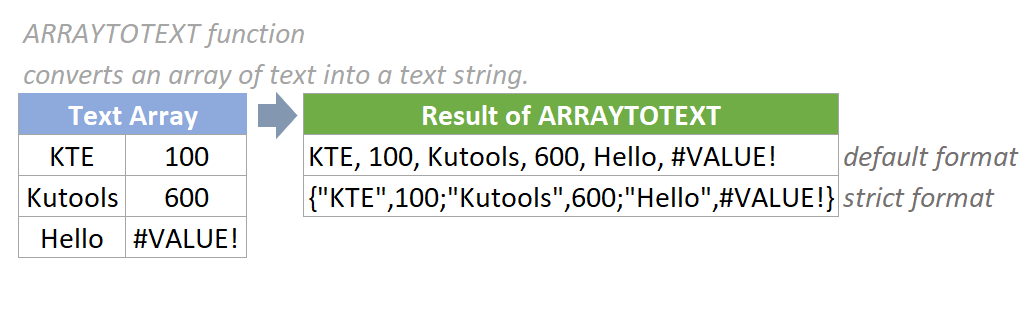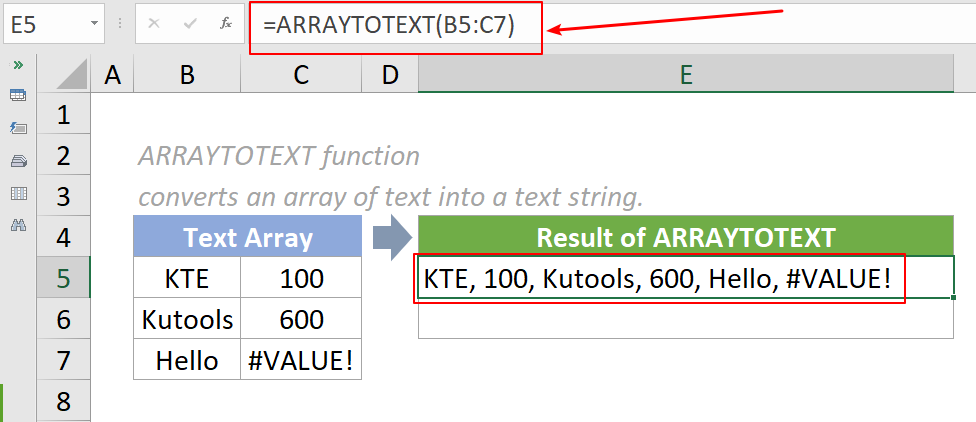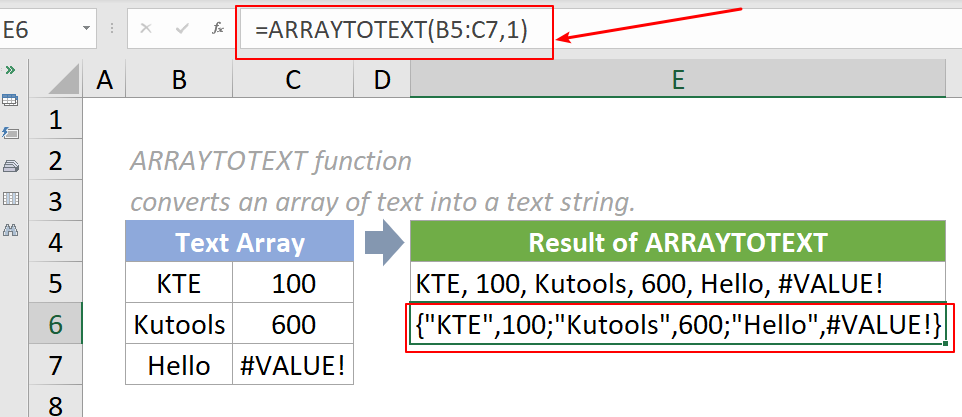## Excel ARRAYTOTEXT Function

The ARRAYTOTEXT Function converts an array or range into a text string.#### Syntax

=ARRAYTOTEXT(array,[format])

#### Arguments

• Array (required): The array to return as a text string.
• Format (optional): The format of the returned text string. It is either 0 (default) or 1.
If it is 0 or omitted, the result format will be concise and easy to read. The text returned will be the same as the text displayed in a cell that has general formatting applied..
If it is 1, the result format will be the strict format including escape characters and row delimiters. The text string will be returned in curly brackets, which can be used directly in the Excel formula bar. Text values will be encapsulated in double quotes (""), and booleans, numbers, and error values are not. Rows are separated by semicolons (;) and columns commas (,).

#### Return Value

The ARRAYTOTEXT function returns a text string.

#### Function notes

1. The ARRAYTOTEXT function is newly introduced in Excel and it is only available in Excel for Microsoft 365 and Excel for the web.
2. The ARRAYTOTEXT function will remove the number formatting applied to any cells containing numeric values.
3. The #VALUE! error value occurs if the format is not 0 or 1.

#### Example

As the below screenshot shows, there is a range of texts listed. To convert them to a text string, please do as follows.

1. To get the text string in concise format, please copy the formula below into cell E5, then press the Enter key to get the result.

=ARRAYTOTEXT(B5:C7)2. To get the text string in strict format, please copy the formula below into cell E6, then press the Enter key to get the result.Note:The format argument in the first formula above is omitted. We can also input the format like the below formula shows:

=ARRAYTOTEXT(B5:C7,0)

#### Relative Functions:

• Excel EVEN Function
The EVEN function rounds numbers away from zero to the nearest even integer.

• Excel EXP Function
The EXP function returns the result of the constant e raised to the nth power.